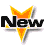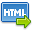Journal :   International Journal of Technology

Volume No. :   4

Issue No. :  1

Year :  2014

Pages :   01-06

ISSN Print :  2231-3907

ISSN Online :  2231-3915Registration

## Numerical Solutions of Mildly Quasi-Linear Hyperbolic Equations and Generalizations

 Address: Urvashi Arora Rajdhani College, Mathematics Department, University of Delhi, Delhi- 110015, India *Corresponding Author
DOI No:

ABSTRACT:
Finite difference methods for the numerical solution of one-space dimensional mildly quasi-linear hyperbolic equation with mixed derivative term, subject to appropriate initial and Dirichlet boundary conditions have been discussed. The methods are three level-implicit finite difference methods of order four. Linear stability analysis and fourth-order approximation at first time level for a one space dimensional quasi-linear hyperbolic equation with non zero second order time derivative term are discussed. The proposed method is generalized for a two and three space dimensional quasi-linear hyperbolic equation with a brief discussion of stability analysis. Numerical results are given to illustrate the accuracy of the proposed methods.
KEYWORDS:
Numerical Solutions of Mildly Quasi-Linear Hyperbolic Equations and Generalizations
Cite:
Urvashi Arora. Numerical Solutions of Mildly Quasi-Linear Hyperbolic Equations and Generalizations; Int. J. Tech. 4(1). Jan.-June. 2014; Page 01-06[View HTML][View PDF]

Visitor's No. :   124742